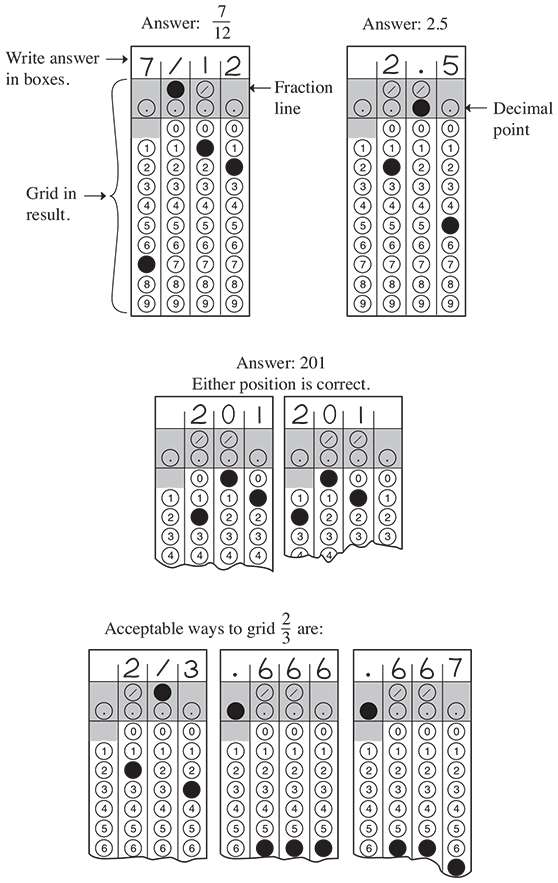Starting October 2015, students will be taking the newly redesigned version of the Preliminary Scholastic Aptitude Test (PSAT). Last month, we discussed the various changes in the test regarding content, scoring, and test format and what it meant for your test prep. This month, we will perform a deep dive into the Math section, an area where many students tend to be the most nervous about. Here we will discuss what to expect on the Math Test questions, what you need to know and how to best prepare to master this section of the PSAT.

## Format

The new PSAT version includes 48 math questions: 40 multiple choice and 8 student-produced responses, also known as grid-ins. The multiple-choice questions will have four possible answers for students to choose from. The grid-in questions require students to calculate and grid the correct answers. In these questions, no answer choices are given. However, some grid-in questions can have more than one correct answer. The Math Test is divided into 2 distinct portions:

 Math Section Time Allotted Number of Questions Avg. Time/Question Math Test – Calculator 45 min 31 ~1 min 27 sec Math Test – No Calculator 25 min 17 ~1 min 28 sec

Yes, you read that correctly. There is a portion of the Math Test where you will be required to use your firsthand knowledge of various math concepts to test your fluency in the subject – and no calculator is allowed. Fluency in the Math Test refers to your ability to quickly solve problems by identifying and using the most efficient solution approaches.

## Why This Information Matters

Are you prepared to solve 17 questions without the use of a calculator? Below is a list of math concepts and operations you’ll need to know how to do by hand:

• Perform basic math skills (addition, subtraction, multiplication, division) using fractions, decimals, and positive &amp negative integers
• Solve algebraic word problems, understand systems of equations & equalities, or algebraic functions
• Supply the correct answer for math problem by thoroughly reading and understanding graphs, charts, diagrams, and tables
• Interpret data and understand the basics of statistics and probability
• Understand geometrical concepts such as area, perimeter and circumference of a triangle, circle or polygon, and parallel and perpendicular lines

## Scoring

Students often wonder the reason behind the various scores received on their PSAT. We understand that it can get a bit confusing. First off, the redesigned PSAT adapted rights-scoring only, which means students receive a point for the correct answer but no deductions for wrong answers. Secondly, the redesigned PSAT will report a total score, section scores, test scores, cross-test scores and subscores. Here is a breakdown of each type of score to help clarify any questions or concerns you may have:

1. Total Score & Section Scores The PSAT will report a Total Score, which is the sum of two Section Scores: Evidence-Based Reading & Writing and Math. Ranges for the Total Score is 320-1520. Ranges for the Section Scores is 160-760.

2. Test Scores The PSAT will report three test scores: the Reading Test score, the Writing & Language Test score and the Math Test score. Ranges for the Test Score is 8-38.

3. Cross-Test Scores The PSAT will report two cross-test scores: Analysis in History/Social Studies and Analysis in Science. These scores, based on questions in the Reading, Writing & Language and Math Tests, asks students to think analytically about texts and problems in these subject areas. Ranges for the Cross-Test Scores is 8-38.

4. Subscores The PSAT will report multiple subscores for Reading, Writing & Language, and Math with a scoring range from 1 to 15 points. Subscores provide more detail about student achievement.

• The Reading Test and the Writing & Language Test each have questions to determine two subscores: Command of Evidence & Words in Context.
• The Writing & Language Test also reports two additional subscores: Expression of Ideas & Standard English Conventions.
• The Math Test reports three subscores: Heart of Algebra, Problem Solving & Data Analysis and Passport to Advanced Math.